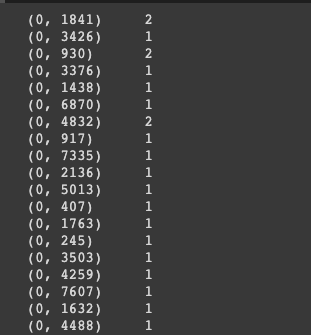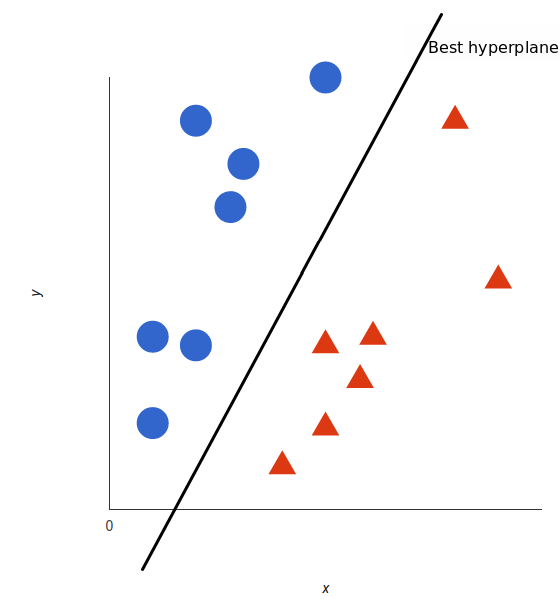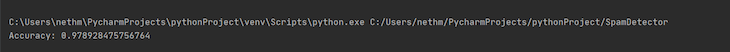Mr. Unity Buddy A game designer and Python lover currently working at ustT Studios.

# Build a machine learning email spam detector with PythonWe’ve all been the recipient of spam emails before. Spam mail, or junk mail, is a type of email that is sent to a massive number of users at one time, frequently containing cryptic messages, scams, or most dangerously, phishing content.

While spam emails are sometimes sent manually by a human, most often, they are sent using a bot. Most popular email platforms, like Gmail and Microsoft Outlook, automatically filter spam emails by screening for recognizable phrases and patterns. A few common spam emails include fake advertisements, chain emails, and impersonation attempts. While these built-in spam detectors are usually pretty effective, sometimes, a particularly well-disguised spam email may fall through the cracks, landing in your inbox instead of your spam folder.

Clicking on a spam email can be dangerous, exposing your computer and personal information to different types of malware. Therefore, it’s important to implement additional safety measures to protect your device, especially when it handles sensitive information like user data.

In this tutorial, we’ll use Python to build an email spam detector. Then, we’ll use machine learning to train our spam detector to recognize and classify emails into spam and non-spam. Let’s get started!

### Prerequisites

First, we’ll import the necessary dependencies. Pandas is a library used mostly used by data scientists for data cleaning and analysis.

Scikit-learn, also called Sklearn, is a robust library for machine learning in Python. It provides a selection of efficient tools for machine learning and statistical modeling, including classification, regression, clustering, and dimensionality reduction via a consistent interface.

Run the command below to import the necessary dependencies:

```import pandas as pd
from sklearn.model_selection import train_test_split
from sklearn.feature_extraction.text import CountVectorizer
from sklearn import svm
```

## Getting started

To get started, first, run the code below:

```spam = pd.read_csv('spam.csv')
```

In the code above, we created a `spam.csv` file, which we’ll turn into a data frame and save to our folder spam. A data frame is a structure that aligns data in a tabular fashion in rows and columns, like the one seen in the following image.

Go ahead and download the sample `.csv` file from GitHub. It mimics the layout of a typical email inbox and includes over 5,000 examples that we’ll use to train our model. It should look like the image below:## Python `train_test_split()`

We’ll use a train-test split method to train our email spam detector to recognize and categorize spam emails. The train-test split is a technique for evaluating the performance of a machine learning algorithm. We can use it for either classification or regression of any supervised learning algorithm.

The procedure involves taking a dataset and dividing it into two separate datasets. The first dataset is used to fit the model and is referred to as the training dataset. For the second dataset, the test dataset, we provide the input element to the model. Finally, we make predictions, comparing them against the actual output.

• Train dataset: used to fit the machine learning model
• Test dataset: used to evaluate the fit of the machine learning model

In practice, we’d fit the model on available data with known inputs and outputs. Then, we’d make predictions based on new examples for which we don’t have the expected output or target values. We’ll take the data from our sample `.csv` file, which contains examples pre-classified into spam and non-spam, using the labels `spam` and `ham`, respectively.

To split the data into our two datasets, we’ll use scikit-learn’s `train_test_split()` method.

Let’s say we have 100 records in the loaded dataset. If we specify the test dataset is 30 percent, we’ll split 70 records for training and use the remaining 30 records for testing.

Run the command below:

```z = spam['EmailText']
y = spam["Label"]
z_train, z_test,y_train, y_test = train_test_split(z,y,test_size = 0.2)
```

`z = spam['EmailText']` assigns the column `EmailText` from spam to `z`. It contains the data that we’ll run through the model. `y = spam["Label"]` assigns the column `Label` from spam to `y`, telling the model to correct the answer. You can see a screenshot of the raw dataset below.

The function `z_train, z_test,y_train, y_test = train_test_split(z,y,test_size = 0.2)` divides columns `z` and `y` into `z_train` for training inputs, `y_train` for training labels, `z_test` for testing inputs, and `y_test` for testing labels.

`test_size=0.2` sets the testing set to 20 percent of `z` and `y`. You can see an example of this in the screenshot below, where the `ham` label indicates non-spam emails, and `spam` represents known spam emails:## Extracting features

Next, we’ll run the code below:

```cv = CountVectorizer()
features = cv.fit_transform(z_train)
```

In `cv= CountVectorizer()`, `CountVectorizer()` randomly assigns a number to each word in a process called tokenizing. Then, it counts the number of occurrences of words and saves it to `cv`. At this point, we’ve only assigned a method to `cv`.

`features = cv.fit_transform(z_train)` randomly assigns a number to each word. It counts the number of occurrences of each word, then saves it to `cv.` In the image below, `0` represents the index of the email. The number sequences in the middle column represent a word recognized by our function, and the numbers on the right indicate the number of times that word was counted:For example, in the image above, the word corresponding to `1841` is used twice in email number `0`.

Now, our machine learning model will be able to predict spam emails based on the number of occurrences of certain words that are common in spam emails.

## Building the model

SVM, the support vector machine algorithm, is a linear model for classification and regression. The idea of SVM is simple, the algorithm creates a line, or a hyperplane, which separates the data into classes. SVM can solve both linear and non-linear problems:Let’s create an SVM model with the code below:

```model = svm.SVC()
model.fit(features,y_train)
```

`model = svm.SVC()` assigns `svm.SVC()` to the model. In the `model.fit(features,y_train)` function, `model.fit` trains the model with `features` and `y_train`. Then, it checks the prediction against the `y_train` label and adjusts its parameters until it reaches the highest possible accuracy.

## Testing our email spam detector

Now, to ensure accuracy, let’s test our application. Run the code below:

```features_test = cv.transform(z_test)
print("Accuracy: {}".format(model.score(features_test,y_test)))
```

The `features_test = cv.transform(z_test)` function makes predictions from `z_test` that will go through count vectorization. It saves the results to the `features_test` file.

In the `print(model.score(features_test,y_test))` function, `mode.score()` scores the prediction of `features_test` against the actual labels in `y_test`.In the image above, you’ll see that we were able to classify spam with 97 percent accuracy.

The full script for this project is below:

```import pandas as pd
from sklearn.model_selection import train_test_split
from sklearn.feature_extraction.text import CountVectorizer
from sklearn import svm

z = spam['EmailText']
y = spam["Label"]
z_train, z_test,y_train, y_test = train_test_split(z,y,test_size = 0.2)

cv = CountVectorizer()
features = cv.fit_transform(z_train)

model = svm.SVC()
model.fit(features,y_train)

features_test = cv.transform(z_test)
print(model.score(features_test,y_test))
```

## Summary

In this tutorial, we learned how to o build and run our model, comparing our predictions against the actual output. Finally, we tested our model using count vectorization.

We’ve only scratched the surface of what we can achieve with machine learning for our email spam detector. We can also add modifications like automating the CSV file or providing a voice assistant.

Hopefully, this article gave you a deeper understanding of some popular machine learning algorithms in Python. Happy coding!Mr. Unity Buddy A game designer and Python lover currently working at ustT Studios.